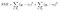# Decision Trees

Tree based models are a part of Non-Parametric Algorithms that are good at dealing with no linear relationships. They are good for Classification and Regression both.

Decision Trees is a Supervised Algorithm .It can be used for both categorical and continuous input and output variables by partitioning the feature space into a number of smaller (non-overlapping) regions with similar response values using a set of splitting rules. It follows a top down approach in aim to find the most optimal decision(feature that best divides the data) at each node based on class labels which is why it is called Greedy Algorithm.

Let’s look at the basic terminology used with Decision trees:

1. Root Node: It represents entire population or sample and this further gets divided into two or more homogeneous sets.
2. Splitting: It is a process of dividing a node into two or more sub-nodes.
3. Decision Node: When a sub-node splits into further sub-nodes, then it is called decision node.
4. Leaf/ Terminal Node: Nodes do not split is called Leaf or Terminal node.

5. Pruning: When we remove sub-nodes of a decision node, this process is called pruning. You can say opposite process of splitting.

6. Branch / Sub-Tree: A sub section of entire tree is called branch or sub-tree.

7. Parent and Child Node: A node, which is divided into sub-nodes is called parent node of sub-nodes where as sub-nodes are the child of parent node.

There are many methodologies for constructing decision trees but the most well-known is the classification and regression tree (CART) algorithm.

CART uses binary recursive partitioning (it’s recursive because each split or rule depends on the the splits above it). The objective at each node is to find the “best” feature (xi) to partition the remaining data into one of two regions (R1 and R2) such that the overall error between the actual response (yi) and the predicted constant (ci) is minimized.

• For REGRESSION problems, the objective function to minimize is the total SSE as defined below:Each Ri is different cluster, so we want to minimize within sum of square error
• For CLASSIFICATION problems, the partitioning is usually made by measuring the impurity of Node. (Impurity occurs when the Terminal Node has more than one class label). Please refer to different impurtiy measures here .

Pruning the Decision Tree: A fully grown tree with large number of nodes is not always desirable because this could overfit to Training data that we definitely do not want. Therefore we Prune the tree to make it shorter and better at predictions Please check this article for complete details.

Blessings

• Great technique for learning models noisy models
• Results are easily interpretable
• Can do automatic stepwise variable selection & complexity reduction
• Robust to outliers and missing data by doing surrogate splits (that would approximate the best fit using another variable
• Fast, simple, and robust
• Decision trees implicitly perform variable screening or feature selection. When we fit a decision tree to a training data set, the top few nodes on which the tree is split are essentially the most important variables within the data set and feature selection is completed automatically

Problems

• Large number of features result in complex trees and could lead to Overfitting
• Greedy Hill climbing algorithm, early bad choice may doom the model
• Pruning may lead to a tree that removes a bad split early but its subtree has a good split later on
• Doesn’t have the best prediction accuracy
• Only makes univariate splits; unable to consider interactions at a given node

Points to Note:

• Re splitting on same feature in two sub branches is a possibility.
• Categorical as well as Numerical Data(by considering average of corresponding observations as a cutoff point for decisions) can be used for splitting.
• Decision Tree is Recursive because it strives to correctly classify members of the population by splitting it into sub-populations based on several dichotomous independent variables.

Problem Solver, Data Science, Actuarial Science, Knowledge Sharer, Hardcore Googler

## More from Shaily jain

Problem Solver, Data Science, Actuarial Science, Knowledge Sharer, Hardcore Googler